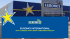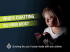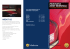# Fractal Pack- educator`s guide

## Transcription

Fractal Pack- educator`s guide
```Fractal Pack 1
Educators’ Guide
Fractals Are SMART: Science, Math & Art!
Fractals Are SMART: Science, Math & Art!
www.FractalFoundation.org
www.FractalFoundation.org
All contents copyright 2009 FractalFoundation
All contents copyright 2009 FractalFoundation
Contents
Introduction
3
Natural Fractals
4
Geometrical Fractals
6
Algebraic Fractals
7
Patterns and Symmetry
8
Ideas of Scale
10
Fractal Applications 11
Fulldome Animations:
12
Crystaloon
Galanga
Pleoria
Morphalingus
Featherino
Peacock
Geometric Fractals
Fractivities:
Sierpinski Triangle Construction
Explore fractals with XaoS
Appendix:
Math & Science Education
Standards met with fractals 16
18
19
Fractals Are SMART: Science, Math & Art!
www.FractalFoundation.org
All contents copyright 2009 FractalFoundation
INTRODUCTION:
WHAT IS A FRACTAL
A fractal is a never ending pattern that repeats itself at different scales.
This property is called “Self-Similarity.”
Fractals are extremely complex, sometimes infinitely complex - meaning you
can zoom in and find the same shapes forever.
Amazingly, fractals are extremely simple to make.
A fractal is made by repeating a simple process again and again.
WHERE DO WE FIND FRACTALS
In Nature
In Geometry
Fractals Are SMART: Science, Math & Art!
www.FractalFoundation.org
All contents copyright 2009 FractalFoundation
In Algebra
NATURAL FRACTALS
BRANCHING
Fractals are found all over nature, spanning a huge range of scales. We find
the same patterns again and again, from the tiny branching of our blood vessels and
neurons to the branching of trees, lightning bolts, and river networks. Regardless of
scale, these patterns are all formed by repeating a simple branching process.
A fractal is a picture that tells the story of the process that created it.
Neurons from the human cortex.
The branching of our brain cells
creates the incredibly complex
network that is responsible for all
we perceive, imagine, remember.
Scale = 100 microns = 10-4 m.
Lichtenberg “lightning”, formed by
rapidly discharging electrons in
lucite. Scale = 10 cm = 10-1 m.
Our lungs are branching fractals with a surface area ~100 m2. The similarity to a tree is
significant, as lungs and trees both use their
large surface areas to exchange oxygen and
CO2. Scale = 30 cm = 3*10-1 m.
Oak tree, formed by a sprout
branching, and then each of the
branches branching again, etc.
Scale = 30 m = 3*101 m.
River network in China, formed by erosion
from repeated rainfall flowing downhill for
millions of years.
Scale = 300 km = 3*105 m.
Fractals Are SMART: Science, Math & Art!
www.FractalFoundation.org
All contents copyright 2009 FractalFoundation
NATURAL FRACTALS
SPIRALS
The spiral is another extremely common fractal in nature, found over a
huge range of scales. Biological spirals are found in the plant and animal kingdoms, and non-living spirals are found in the turbulent swirling of fluids and in the
pattern of star formation in galaxies.
All fractals are formed by simple repetition, and combining expansion and
rotation is enough to generate the ubiquitous spiral.
A fossilized ammonite from
300 million years ago. A
simple, primitive organism, it
built its spiral shell by adding
pieces that grow and twist at a
constant rate. Scale = 1 m.
A hurricane is a self-organizing spiral in
the atmosphere, driven by the evaporation
and condensation of sea water.
Scale = 500 km = 5*105 m.
The plant kingdom is full of spirals. An
agave cactus forms its spiral by growing
new pieces rotated by a fixed angle. Many
other plants form spirals in this way, including sunflowers, pinecones, etc.
Scale = 50 cm = 5*10-1 m.
A spiral galaxy is the largest natural
spiral comprising hundreds of billions
of stars. Scale = 100,000 ly = ~1020 m.
The turbulent motion of fluids creates spirals in systems ranging
from a soap film to the oceans,
atmosphere and the surface of jupiter. Scale = 5 mm = 5*10-3 m.
Fractals Are SMART: Science, Math & Art!
www.FractalFoundation.org
All contents copyright 2009 FractalFoundation
A fiddlehead fern is a self-similar
plant that forms as a spiral of
spirals of spirals.
Scale = 5 cm = 5*10-2 m.
GEOMETRIC FRACTALS
Purely geometric fractals can be made by repeating a simple process.
The Sierpinski Triangle is made by repeatedly removing the middle
triangle from the prior generation. The number of colored triangles increases
by a factor of 3 each step, 1,3,9,27,81,243,729, etc.
See the Fractivity on page 15 to learn to teach elementary school
students how to draw and assemble Sierpinski Triangles.
The Koch Curve is made by repeatedly replacing each segment of a generator
shape with a smaller copy of the generator. At each step, or iteration, the total length
of the curve gets longer, eventually approaching infinity. Much like a coastline, the
length of the curve increases the more closely you measure it.
Fractals Are SMART: Science, Math & Art!
www.FractalFoundation.org
All contents copyright 2009 FractalFoundation
ALGEBRAIC FRACTALS
We can also create fractals by repeatedly calculating a simple equation
over and over. Because the equations must be calculated thousands or millions
of times, we need computers to explore them. Not coincidentally, the Mandelbrot
Set was discovered in 1980, shortly after the invention of the personal computer.
HOW DOES THE MANDELBROT SET WORK
We start by plugging a value for the variable ‘C’ into the simple equation
below. Each complex number is actually a point in a 2-dimensional plane. The
equation gives an answer, ‘Znew’ . We plug this back into the equation, as ‘Zold’
and calculate it again. We are interested in what happens for different starting
values of ‘C’.
Generally, when you square a number, it gets bigger, and then if you
square the answer, it gets bigger still. Eventually, it goes to infinity. This is the
fate of most starting values of ‘C’. However, some values of ‘C’ do not get bigger,
but instead get smaller, or alternate between a set of fixed values. These are the
points inside the Mandelbrot Set, which we color black. Outside the Set, all the
values of ‘C’ cause the equation to go to infinity, and the colors are proportional to
the speed at which they expand.
The interesting places in ths fractal are all on the edge. We can zoom in forever,
and never find a clear edge. The deeper we explore, the longer the numbers become,
and the slower the calculations are. Deep fractal exploration takes patience!
Fractals Are SMART: Science, Math & Art!
www.FractalFoundation.org
All contents copyright 2009 FractalFoundation
PATTERNS & SYMMETRY
The great value of fractals for education is that they make abstract math visual.
When people see the intricate and beautiful patterns produced by equations, they lose
their fear and instead become curious.
Z2
Z3
Z4
Z5
Exploring fractals is fun, and we can play with the equations to see what happens. The 4
images above are algebraic fractals known as Julia Sets. The first image in the upper left
comes from the same equation as the Mandelbrot Set, Z = Z2 + C. When we raise the exponent to Z3 (i.e. Z*Z*Z), the Julia Set takes on a 3-fold symmetry, and so on. The degree
of symmetry always corresponds to the degree of the exponent.
Fractals Are SMART: Science, Math & Art!
www.FractalFoundation.org
All contents copyright 2009 FractalFoundation
PATTERNS & SYMMETRY
Just as we find branching fractals in nature, we also find branching within algebraic
fractals like the Mandelbrot Set. Known as “Bifurcation”, branching in these fractals is a
never-ending process. The four images below are successive zooms into a detail of the
Z = Z2 + C Mandelbrot Set. Two-fold symmetry branches and becomes 4-fold, which
doubles into 8-fold, and then 16-fold. The branching process continues forever, and the
number of arms at any level is always a power of 2.
If we explore other algebraic fractals, we find similar patterns and progressions.
The two images below are details from the Z = Z3 + C Mandelbrot Set. Since the equation
involves Z cubed, the arms now branch in 3-fold symmetry. Each of the 3 arms branches
into 3 more arms, becoming 9-fold symmetry. This then trifurcates into 27 arms, 81, 243,
etc. where the number of arms is always a power of 3.
Again, the educational value of fractals is that they make the behavior of equations visible.
Zooming into fractals, math ceases to be intimidating, and instead becomes entrancing.
Fractals Are SMART: Science, Math & Art!
www.FractalFoundation.org
All contents copyright 2009 FractalFoundation
IDEAS OF SCALE
HOW BIG (OR SMALL) ARE FRACTALS
Mathematical fractals are infinitely complex. This means we can zoom into
them forever, and more detail keeps emerging. To describe the scale of fractals,
we must use scientific notation:
Thousand
Million
Billion
Trillion
1,000
1,000,000
1,000,000,000
1,000,000,000,000 1,000,000,000,000,000
103
106
109
1012
1015
Because of the limits of computer processors, all the fulldome fractal zooms stop
at a magnification of 1016. Of course the fractals keep going, but it becomes much slower
to compute deeper than that. 1016 (or ten quadrillion) is incredibly deep. To put it in
perspective, the diameter of an atom is about 10-10 meters, so as we zoom six orders of
magnitude smaller, we’re looking at things a million times smaller than an atom!
Or, to look at it another way, as we zoom into the fractals, the original object keeps growing.
How big does it get when we have zoomed in 1016 times? The orbit of the dwarf planet
Pluto is about 1012 meters in diameter. If we start zooming in a 10 meter dome, then the
original image grows to a size larger than our entire solar system - 100,000 times larger!
All of these zooms are just scratching the surface of the infinitely complex.
Some fractals, like the Mandelbrot Set, become even more intricate and beautiful
the deeper we explore. The image above exists at a depth of 10176 magnification!
Fractals Are SMART: Science, Math & Art!
www.FractalFoundation.org
All contents copyright 2009 FractalFoundation
FRACTAL APPLICATIONS
A commonly asked question is: What are fractals useful for
Nature has used fractal designs for at least hundreds of millions of years.
Only recently have human engineers begun copying natural fractals for inspiration
to build successful devices. Below are just a few examples of fractals being used
in engineering and medicine.
A computer chip cooling circuit etched in
a fractal branching pattern. Developed by
researchers at Oregon State University, the
device channels liquid nitrogen across the
surface to keep the chip cool.
Researchers at Harvard Medical School and
elsewhere are using fractal analysis to assess
the health of blood vessels in cancerous tumors.
Fractal analysis of CT scans can also quantify
the health of lungs suffering from emphysema or
other pulmonary illnesses.
Fractal antennas developed by Fractenna
in the US and Fractus in Europe are making
their way into cellphones and other devices.
Because of their fractal shapes, these antennas can be very compact while receiving
radio signals across a range of frequencies.
Amalgamated Research Inc (ARI) creates
space-filling fractal devices for high precision
fluid mixing. Used in many industries, these
devices allow fluids such as epoxy resins to
be carefully and precisely blended without the
need for turbulent stirring.
Fractals Are SMART: Science, Math & Art!
www.FractalFoundation.org
All contents copyright 2009 FractalFoundation
FULLDOME ANIMATIONS
FRACTAL PACK 1
CRYSTALOON
This fractal animation explores
the Mandelbrot Set, the archetypal
algebraic fractal. It comes from the
very simplest possible non-linear
equation:
We journey into a region known as the “Crystal Canyon”, due to the crystalline
nature of the decorations. Zooming into one of the jewels along the edge, the journey
progresses into a wheel of mesh-like crystals. The zoom continues into a sequence
of self-similar units before veering into the connection point between units. At this
point the patterns begin to bifurcate, turning from 2-fold to 4-fold symmetry, then 8, 16,
32, etc, all surrounding a miniature replica of... the entire Mandelbrot Set. The zoom
culminates at a magnification of 1016, or a million times smaller than the diameter of a
hydrogen atom.
GALANGA
This zoom explores the “Snowflake
Village,” another region within the
Mandelbrot Set. One of the astonishing
perfectly self-similar. Different areas have
vastly different structural styles, and the
entire Set contains an infinite variety of
shapes and patterns. Another amazing discovery about
the Mandelbrot Set is that this purely
abstract entity contains structures that closely resemble the fractals of nature.
In Galanga, we find objects reminiscent of snowflakes, flowers, pine trees, bacteria,
chromosomes, insects, etc - all created from the extremely simple equation above.
Fractals Are SMART: Science, Math & Art!
www.FractalFoundation.org
All contents copyright 2009 FractalFoundation
Pleoria
In this animation, we are using the
same equation, Z = Z2 + C but we plot the
inverse of the values, which causes the
whole Set to turn dramatically inside out.
This zoom explores the details around the
edges of the miniature embedded Mandelbrot replicas, which give rise to structures
we refer to as virtual Julia Sets. We encounter structures reminiscent of lightning
bolts, brains and flowers.
MORPHALINGUS
In this animation, we start with the
same general formula for the Mandelbrot
Set, but with an additional complex term
added in. This results in a similar fractal,
but with some very different characteristics. The zoom explores several different
areas of the fractal, each of which has its
own distinct musical accompaniment.
Fractals Are SMART: Science, Math & Art!
www.FractalFoundation.org
All contents copyright 2009 FractalFoundation
FEATHERINO
In this animation, we are exploring
the behavior of a very different equation:
This equation was actually created
over 300 years ago by Isaac Newton,
and it describes the process known as
Newton’s Method for solving a polynomial equation. Of course, without a computer,
Newton could not see how beautiful his equation really is. The fractal created by this
equation is perfectly self-similar, and it is infinitely large as well as infinitely small.
PEACOCK
This fractal comes from yet another
equation:
While this fractal equation is related to
the Newton fractal above, it behaves
very differently. It is not perfectly self-similar, but instead it gains in complexity as we
zoom in. Further, embedded within this fractal, we find tiny copies of the Mandelbrot Set!
Fractals Are SMART: Science, Math & Art!
www.FractalFoundation.org
All contents copyright 2009 FractalFoundation
GEOMETRIC FRACTALS
In this animation, we explore
fractals that are made using simple
geometric repetition of shapes rather
than equations. We can watch trees
branch and grow in 3-dimensions, ferns
unfurl, and a host of abstract fractal
shapes come into being, swirl about
and dissolve into clouds of fractal dust.
This animation lets us see how
simple it really is to grow fractals, and it
helps us understand how the incredible
complexity of natural forms all around
us comes about by simple repetition.
Fractals Are SMART: Science, Math & Art!
www.FractalFoundation.org
All contents copyright 2009 FractalFoundation
Fractals Are SMART: Science, Math & Art!
www.FractalFoundation.org
All contents copyright 2009 FractalFoundation
Fractals Are SMART: Science, Math & Art!
www.FractalFoundation.org
All contents copyright 2009 FractalFoundation
Fractivity: Explore Fractals with XaoS
First, download and install the XaoS program (either Mac or Windows version) from:
http://fractalfoundation.org/resources/fractal-software/
When you run the program, it opens with an image of the Mandelbrot set.
To navigate: just point the mouse and click! On a PC, the left button zooms in and the
right zooms out. On a Mac, use ctrl-click to zoom out. To pan the image around, use
both buttons together, or shift-click on the Mac.
Set the defaults: From the ‘Filters’ menu, enable Palette Emulator. From the
“Calculation” menu select Iterations, and raise it to 2000. From the ‘File’ menu, select
Save Configuration so you don’t have to make these changes again.
Color palettes are randomly generated, and can be changed with the “P” key. To cycle
the colors, use “Y”. There are many filters and effects to explore from the menus.
XaoS can create many different fractal types, which can be accessed by using
the number keys:
Keys 1 to 5 are Mandelbrot sets with various powers. The “normal” X^2 Mandelbrot
set is on key 1. (Hitting “1” is a good way to reset yourself if you get lost!)
Key 6 is a Newton fractal, exponent 3, illustrating Newton’s method for finding roots to
3’d order polynomial equations.
Key 7 is the Newton fractal for exponent 4.
Key 8,9, and 0 are Barnsley fractals.
Key A - N are several other fascinating fractal formulas
Julia Sets: Every point in the Mandelbrot set (and several of the other fractals)
corresponds to a unique Julia set. To explore the relationship between the Mandelbrot
and Julia fractals, press “J” to enter fast-Julia mode. When you find a Julia set you
like, switch over to it by pressing “M”.
To save a fractal, use “File->Save Image” to save the picture for use in other
prgrams. Use “File->Save” to save the actual parameters of the file, which will allow
you to return to the fractal in XaoS and keep exploring it further.
Finally - use the Help file and explore the excellent tutorials! Though written by
Jan Hubicka - the initial programmer - originally in Czech, they are very useful both to
learn how to use the program as well as to learn about the fractals. Enjoy!
Fractals Are SMART: Science, Math & Art!
www.FractalFoundation.org
All contents copyright 2009 FractalFoundation
SCIENCE AND MATH
EDUCATIONAL STANDARDS
From the National Council of Teachers of Mathematics (NCTM):
Recognize geometric shapes and structures in the environment and specify their
location. (NCTM Geometry grades K-2)
Recognize and apply slides, flips, and turns; recognize and create shapes that
have symmetry. (NCTM Geometry grades K-2)
Investigate, describe, and reason about the results of subdividing, combining, and
transforming shapes. (NCTM Geometry grades 3-5)
Identify and describe line and rotational symmetry in two- and three-dimensional
shapes and designs. (NCTM Geometry grades 3-5)
Recognize and apply geometric ideas and relationships in areas outside the mathematics classroom, such as art, science, architecture, and everyday life.
(NCTM Geometry grades 6-8; 9-12)
Identify functions as linear or nonlinear and contrast their properties from tables,
graphs, or equations. (Grades 6-8 NCTM Expectations for Algebra Knowledge)
Use symbolic expressions, including iterative and recursive forms, to represent
relationships arising from various contexts. (Grades 9-12 NCTM Expectations for
Algebra Knowledge)
From the National Science Education Standard; National Academy of Sciences:
UNIFYING CONCEPTS AND PROCESSES STANDARD:
As a result of activities in grades K-12, all students should develop understanding
and abilities aligned with the following concepts and processes:
•
Systems, order, and organization
•
Evidence, models, and explanation
•
Constancy, change, and measurement
•
Evolution and equilibrium
•
Form and function
Structure and function in living systems; Diversity and adaptations of organisms;
Interdependence of organisms.
Fractals Are SMART: Science, Math & Art!
www.FractalFoundation.org
All contents copyright 2009 FractalFoundation
```

### Fractals, Dynamical Systems and Chaos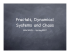### Creating Fractals - MyopicBadger Fractals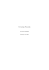### Exploring the Effect of Direction on Vector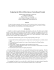### Slides of the fifth lecture (Fractals and multifractals, 4739KB)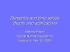### Fractals and 3D printing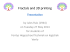### Chapter 8 Fractal properties of plants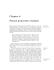### (Microsoft PowerPoint - La complessit\340 della realt\340 biologica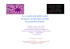### Teacher Resource Packet - Culturally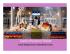### Genuine Fractals 4.1 Evaluation Guide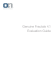### Size and Shape, Scale and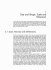### our faculty hiring brochure### Look. Donʼt touch.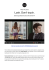### shared branching - Frontier Financial Credit Union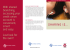### Fractal Dimension in Butterflies` Wings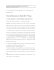### smart healthcare consumer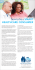### EURONICS INTERNATIONAL